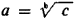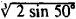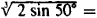# Taking The Logarithm

The following article is from The Great Soviet Encyclopedia (1979). It might be outdated or ideologically biased.

## Logarithm, Taking The

the operation of finding the logarithm of a numerical, algebraic, or other expression. Taking the logarithm is one of two operations that are the inverse of raising to a power. If ab = c, thenand b = logac. Taking the logarithm is used in practical computations to reduce the operations of multiplication, division, raising to a power, and extraction of a root to the operations of addition, subtraction, multiplication, and division. For example, in order to approximatewe may use the relationship log(1/3) log 2 + (1/3) log sin 50° and then tables of logarithms.

The Great Soviet Encyclopedia, 3rd Edition (1970-1979). © 2010 The Gale Group, Inc. All rights reserved.
Mentioned in ?
References in periodicals archive ?
that is experimentally measured by adding the molecule to an octanol/water mixture (OW) and by taking the logarithm of the ratio [[K.sub.(OW)]] of the amount that dissolves in the octanol phase to the amount that dissolves in the water phase.
Formula (1) belongs to a linearized nonlinear time-varying model, replacing variables into multiple linear time-varying models and by taking the logarithm from formula (1) on both sides it can be transformed into
And for handling nonlinear data fitting problems, the least squares method could only approximate it into a linear problem by taking the logarithm of both ends which resulted in migration of the optimal solution .
Taking the logarithm of both sides of (1.4) and using Mobius inversion gives (see [BLL98]) the following refinement of (1.2),
Taking the logarithm of both sides of Equation 5, Equation 6 is obtained,
For isothermal conditions, taking the logarithm of Eq 4 gives:
Taking the logarithm shows that a double logarithmic plot yields straight lines.
By taking the logarithm in Stirling's Formula, [lim.[n[right arrow][infinity]]] [n.summation over (k=1)] ln k - n(ln n) - 1/2(ln n) + n] = 1/2[ln(2[pi])].
Substituting the last expression into equation (1) and taking the logarithm of both sides, one obtains:
Taking the logarithm on both sides of (2), it leads to a linear relation between the ln [??] and ln [sigma] for a constant loading temperature:
Taking the logarithm derivative from both sides of (22) we get

Site: Follow: Share:
Open / Close